Halstead (1977) distinguishes software science from computer science. The premise of software science is that any programming task consists of selecting and arranging a finite number of program "tokens," which are basic syntactic units distinguishable by a compiler. A computer program, according to software science, is a collection of tokens that can be classified as either operators or operands. The primitive measures of Halstead's software science are: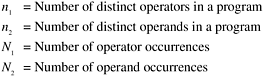Based on these primitive measures, Halstead developed a system of equations expressing the total vocabulary, the overall program length, the potential minimum volume for an algorithm, the actual volume (number of bits required to specify a program), the program level (a measure of software complexity), program difficulty, and other features such as development effort and the projected number of faults in the software. Halstead's major equations include the following:

 Vocabulary ( n )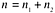Length ( N )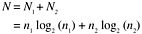Volume ( V )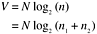Level ( L )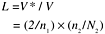Difficulty ( D ) (inverse of level)Effort (E)Faults ( B )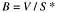where V * is the minimum volume represented by a built-in function performing the task of the entire program, and S * is the mean number of mental discriminations (decisions) between errors ( S * is 3,000 according to Halstead).

Halstead's work has had a great impact on software measurement. His work was instrumental in making metrics studies an issue among computer scientists. However, software science has been controversial since its introduction and has been criticized from many fronts. Areas under criticism include methodology, derivations of equations, human memory models, and others. Empirical studies provide little support to the equations except for the estimation of program length. Even for the estimation of program length, the usefulness of the equation may be subject to dispute. To predict program length, data on N 1 and N 2 must be available, and by the time N 1 and N 2 can be determined, the program should be completed or near completion. Therefore, the predictiveness of the equation is limited. As discussed in Chapter 3, both the formula and actual LOC count are functions of N 1 and N 2 ; thus they appear to be just two operational definitions of the concept of program length. Therefore, correlation exists between them by definition.

In terms of quality, the equation for B appears to be oversimplified for project management, lacks empirical support, and provides no help to software engineers . As S * is taken as a constant, the equation for faults ( B ) simply states that the number of faults in a program is a function of its volume. This metric is therefore a static metric, ignoring the huge variations in fault rates observed in software products and among modules.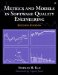Metrics and Models in Software Quality Engineering (2nd Edition)
ISBN: 0201729156
EAN: 2147483647
Year: 2001
Pages: 176